No related product information!

# Lesson 6How to ControlDC Motor

In this lesson, we will learn how to control DC Motor.

6.1 Components used in this course

 Components Quantity Picture Raspberry Pi 1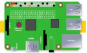Motor HAT 1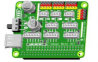DC Motor 1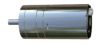6.2 The introduction of DC Motor

PiCar-b robot products use DC motor as a power device. DC motor is a device that converts DC electrical energy into mechanical energy. It is widely used to drive various equipment, such as electric fans, remote control cars, electric windows, etc.The DC motor is very suitable as the walking mechanism of the robot.

6.3 Wiring diagram (Circuit diagram)

When the DC Motor module is in use, it needs to be connected to the motorA. interface on the Motor HAT drive board. Use the motor cable to connect, the large connector is connected to the HAT, and the small connector is connected to the motor.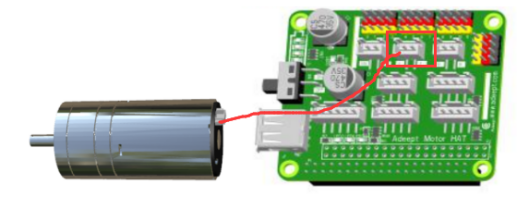6.4 How to control Motor

### Run the code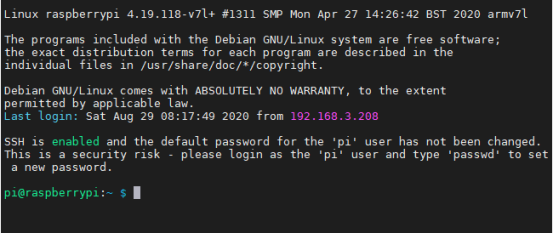2. Enter the command and press Enter to enter the folder where the program is located: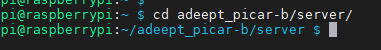3. View the contents of the current directory file:

ls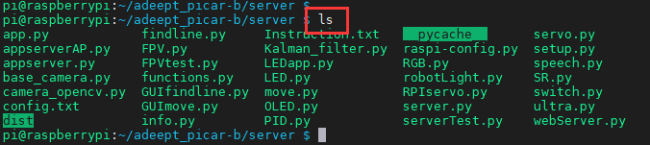4. Enter the command and press Enter to run the program:

sudo python3 move.py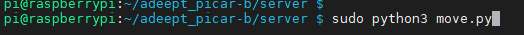5. After running the program successfully, you will observe that the Motor will rotate for about 1 second and then stop, and the program will also stop. If you need the motor to rotate again, you need to run the program again.

6.5 The main code program of this lesson

For the complete code, please refer to the file move.py.

 1. import time  2. import RPi.GPIO as GPIO  3.   4. Motor_A_EN    = 4  5. Motor_A_Pin1  = 26  6. Motor_A_Pin2  = 21

Import the dependent library, where Motor_EN = 4, Motor_Pin1 = 26, Motor_Pin2 = 21 are the parameters of the corresponding interface motorA.

 1. def setup():#Motor initialization  2.     global pwm_A  3.     GPIO.setwarnings(False)  4.     GPIO.setmode(GPIO.BCM)  5.     GPIO.setup(Motor_A_EN, GPIO.OUT)  6.     GPIO.setup(Motor_A_Pin1, GPIO.OUT)  7.     GPIO.setup(Motor_A_Pin2, GPIO.OUT)  8.   9.     motorStop()  10.     try:  11.         pwm_A = GPIO.PWM(Motor_A_EN, 1000)  12.     except:  13.         pass

Set the motor initialization function.

 1. def destroy():  2.     motorStop()  3.     GPIO.cleanup()             # Release resource  4.

Set the motor stop function.

 1. def move(speed, direction, turn, radius=0.6):   # 0 < radius <= 1    2.     #speed = 100  3.     if direction == 'forward':  4.         if turn == 'right':  5.             motor_left(0, left_backward, int(speed*radius))  6.             motor_right(1, right_forward, speed)  7.         elif turn == 'left':  8.             motor_left(1, left_forward, speed)  9.             motor_right(0, right_backward, int(speed*radius))  10.         else:  11.             motor_left(1, left_forward, speed)  12.             motor_right(1, right_forward, speed)  13.     else:  14.         pass

Set the motor rotation function.

 1. if __name__ == '__main__':  2.     try:  3.         speed_set = 60  4.         setup()  5.         move(speed_set, 'forward', 'no', 0.8)  6.         time.sleep(1.3)  7.         motorStop()  8.         destroy()  9.     except KeyboardInterrupt:  10.         destroy()

Instantiate the object.